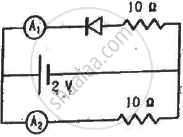Advertisement Remove all ads

# What Are the Readings of the Ammeters A1 And A2 Shown in Figure. Neglect the Resistance of the Meters. - Physics

Short Note

What are the readings of the ammeters A1 and A2 shown in figure. Neglect the resistance of the meters.(Assume that the resistance of each diode is zero in forward bias and is infinity in reverse bias.)

Advertisement Remove all ads

#### Solution

From the circuit diagram, we can see that one diode (the upper one) is reverse biassed.
We know that when a diode is forward biassed, it has zero resistance ideally. So, it can be replaced by a short circuit. When a diode is reverse biassed, it has infinite resistance ideally. So, it can be replaced by an open circuit.
Thus,
In the given circuit, one diode is reverse biassed. So, it can be replaced by an open circuit. Hence, the current in this branch will be zero.
So, the current through A1 is zero.
For A2
Current =  $\frac{2}{10}$  = 0.2 A

Concept: p-n Junction
Is there an error in this question or solution?
Advertisement Remove all ads

#### APPEARS IN

HC Verma Class 11, Class 12 Concepts of Physics Vol. 2
Chapter 23 Semiconductors and Semiconductor Devices
Q 26 | Page 420
Advertisement Remove all ads
Advertisement Remove all ads
Share
Notifications

View all notifications

Forgot password?